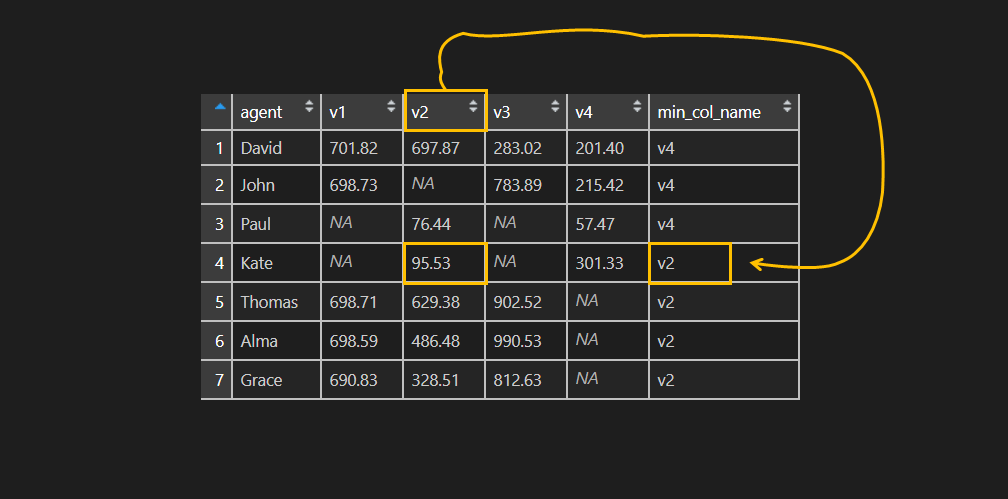# Combine apply and match in R, return the name of the column

Here is how to use functions apply and match together in R and a result – return the name of the column that contains minimum or maximum.

Here is an example about using apply to calculate minimum or maximum for each row of the data frame.

How about the column name that contains this value?

When you understand how to use other functions to apply, it is easy.

Here is my data frame.

```df <- data.frame(
agent = as.character(c("David", "John", "Paul", "Kate", "Thomas", "Alma", "Grace")),
v1 = as.numeric(c(701.82, 698.73, NA, NA, 698.71, 698.59, 690.83)),
v2 = as.numeric(c(697.87, NA, 76.44, 95.53, 629.38, 486.48, 328.51)),
v3 = as.numeric(c(283.02, 783.89, NA, NA, 902.52, 990.53, 812.63)),
v4 = as.numeric(c(201.40, 215.42, 57.47, 301.33, NA, NA, NA))
)

df

#   agent     v1     v2     v3     v4
#1  David 701.82 697.87 283.02 201.40
#2   John 698.73     NA 783.89 215.42
#3   Paul     NA  76.44     NA  57.47
#4   Kate     NA  95.53     NA 301.33
#5 Thomas 698.71 629.38 902.52     NA
#6   Alma 698.59 486.48 990.53     NA
#7  Grace 690.83 328.51 812.63     NA```

I would like to return the column name that matches the minimum value in a relevant row. In this example, I should use only from the second to the fifth column. Function min will return, but I should prepare to deal with NA values with argument na.rm. Match will return the position of that value in the row vector. Apply function will execute calculations in each row is by using margin parameter 1.

### Apply and match in R

Together apply and match in R looks like this.

```df\$min_col_name <-
names(df[2:5])[
apply(df[2:5], MARGIN = 1, function(x) match(min(x, na.rm = T), x)
)]

df

#   agent     v1     v2     v3     v4 min_col_name
#1  David 701.82 697.87 283.02 201.40           v4
#2   John 698.73     NA 783.89 215.42           v4
#3   Paul     NA  76.44     NA  57.47           v4
#4   Kate     NA  95.53     NA 301.33           v2
#5 Thomas 698.71 629.38 902.52     NA           v2
#6   Alma 698.59 486.48 990.53     NA           v2
#7  Grace 690.83 328.51 812.63     NA           v2```

### Here are additional examples with the use of the apply family

How to combine files with R and add filename column

How to combine numbers and find sums with R

Check if a column has a missing values (NA) in R

Categories R